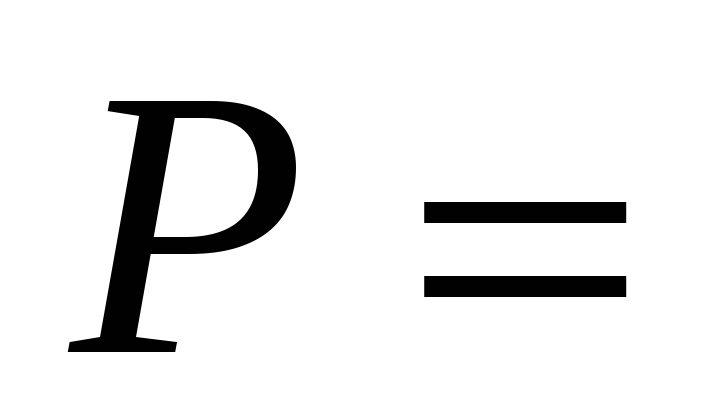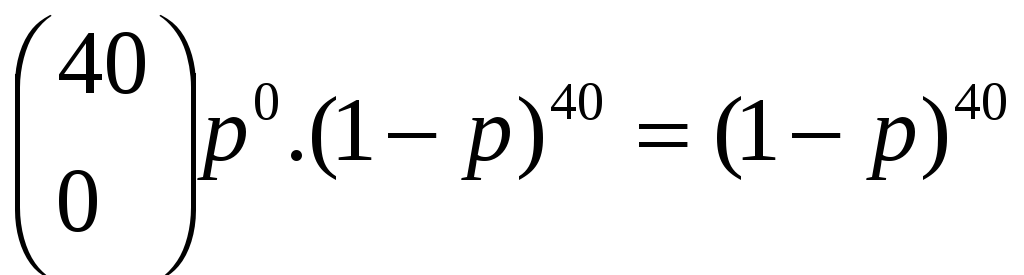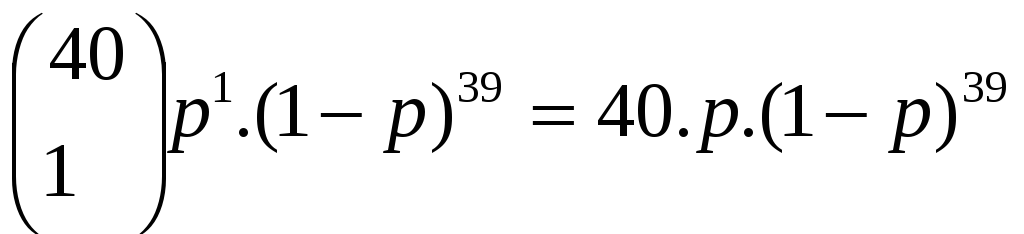# Example Question

 Example Question: Consider the HEC mechanism. Let p be the probability that a bit is received in error. With what probability a cell is rejected when the HEC state machine is in the "Correction Mode"? With what probability a cell is rejected when the HEC state machine is in the "Detection Mode"? Assume that the HEC state machine is in the "Correction Mode." What is the probability that n successive cells will be rejected, where n >= 1 ? Assume that the HEC state machine is in the "Correction Mode." What is the probability p(n) that n successive cells will be accepted, where n >= 1 ? Hint: Write down the expression for p(1) and p(2), and express p(3) as a function of p(1) and p(2). Then write down the general expression for p(n) for any n as a function of p(n-1) and p(n-2). Answer: p = probability that a bit is erroneous. A cell is rejected if it has multiple-bit error in its header, when the HEC state machine is the “Correction Mode”. In other words, for a cell not to be discarded, it should have at most 1-bit error in its header. The header is 40 bits-long. Hence, P = probability of a cell is rejected when HEC state machine is in the “Correction Mode” can be computed as follows;1-p(no bit error in header)-p(1 bit error in header) p(no bit error)=p(1-bit error)=Share with your friends: